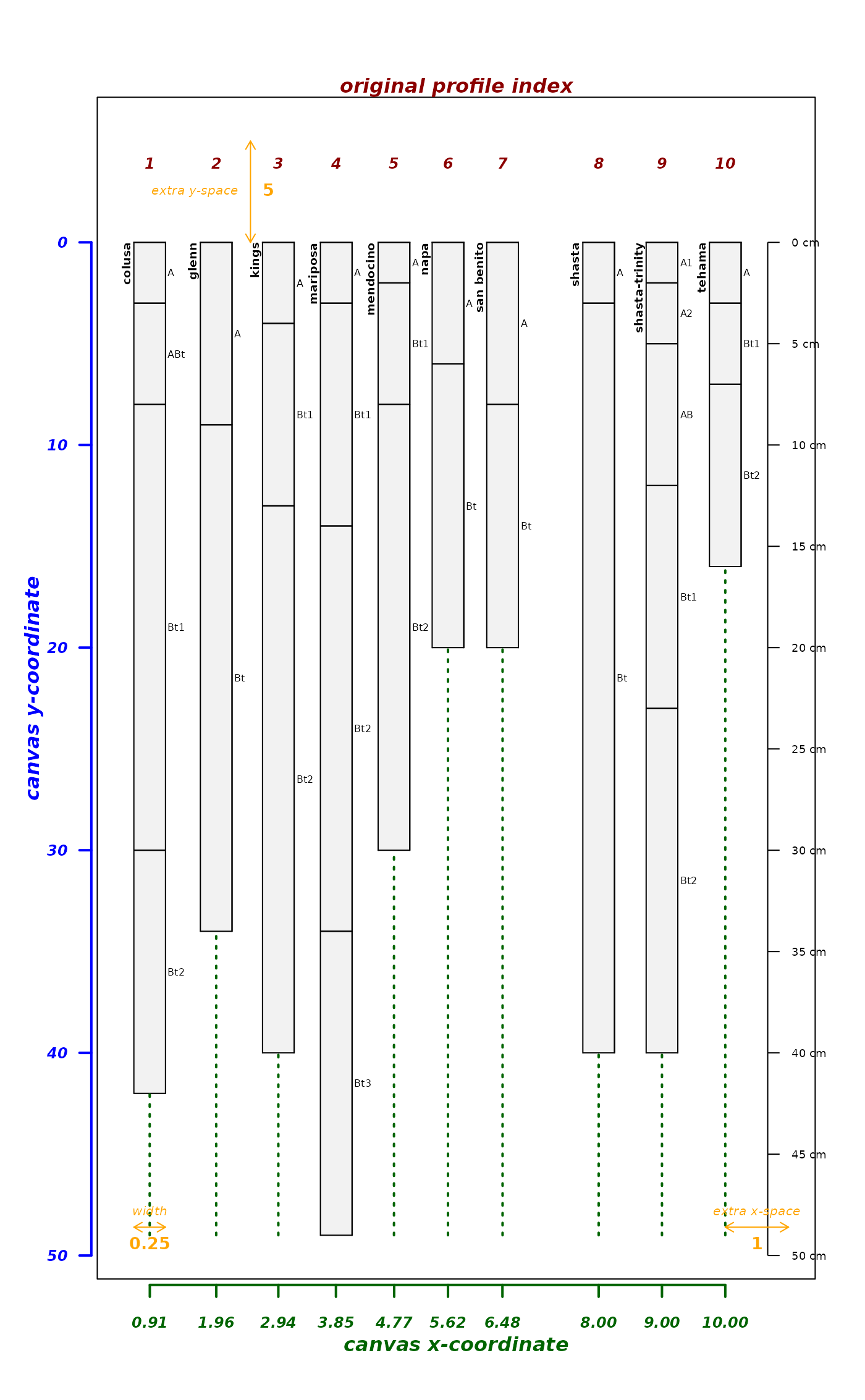Create a visual explanation for the many arguments to plotSPC. Call this function instead of plotSPC, all objects after x are passed on to plotSPC. Nearly all of the figures in the Introduction to SoilProfileCollection Objects tutorial are created with this function.

explainPlotSPC(x, ...)

## Arguments

x

a SoilProfileCollection object

...

arguments passed to plotSPC

## Value

a list of internally-used ordering vectors and graphical offsets / scaling factors

plotSPC

D.E. Beaudette

## Examples


# sample data
data(sp4)
depths(sp4) <- id ~ top + bottom

# proposed vector of relative positions, overlap likely
pos <- c(1, 1.1, 3, 4, 5, 5.2, 7, 8, 9, 10)

# try it
explainPlotSPC(sp4, name='name', relative.pos=pos)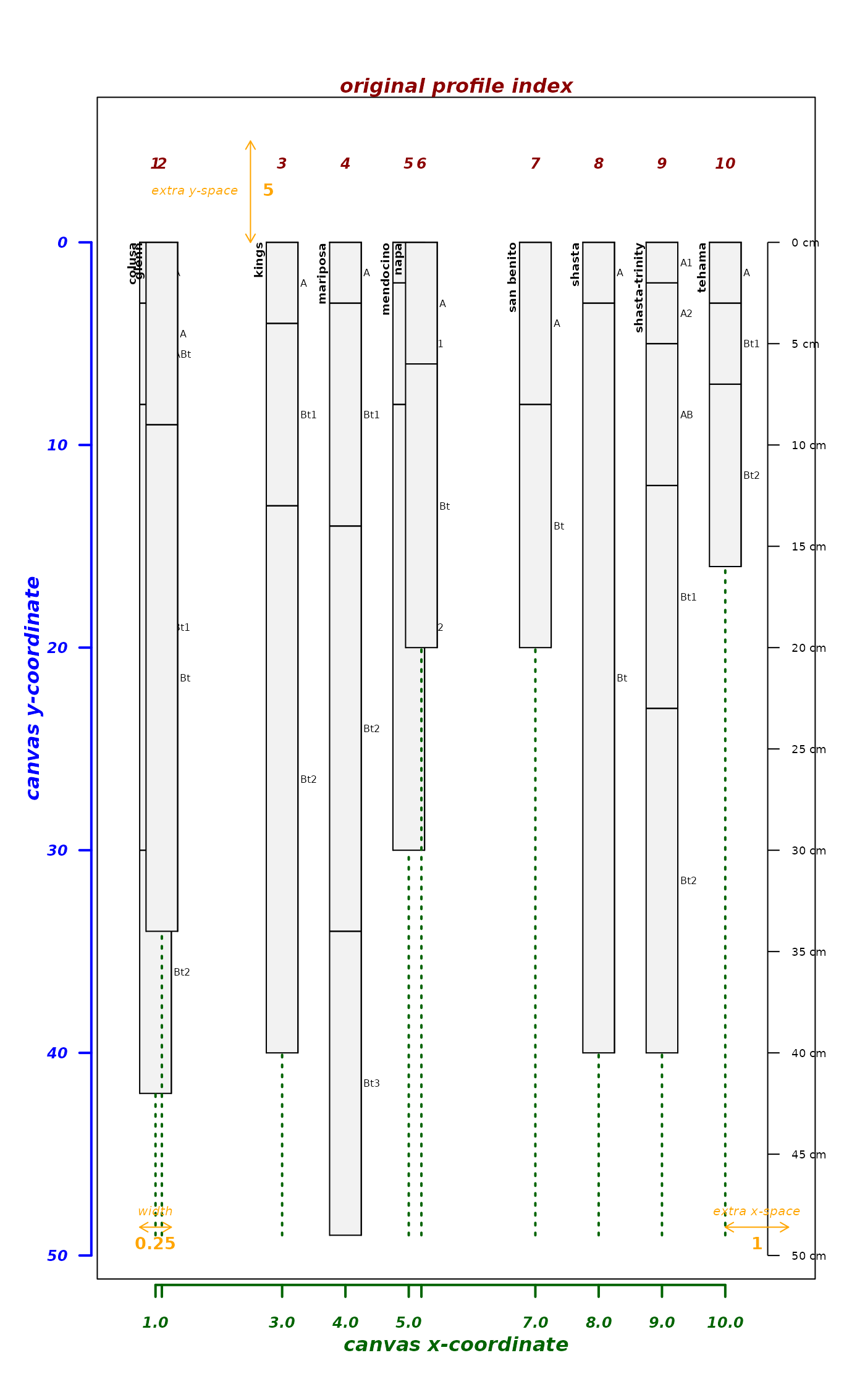# attempt to fix using an integer sequence, short-circut will prevent adjustments
explainPlotSPC(sp4, name='name', relative.pos=fixOverlap(1:10))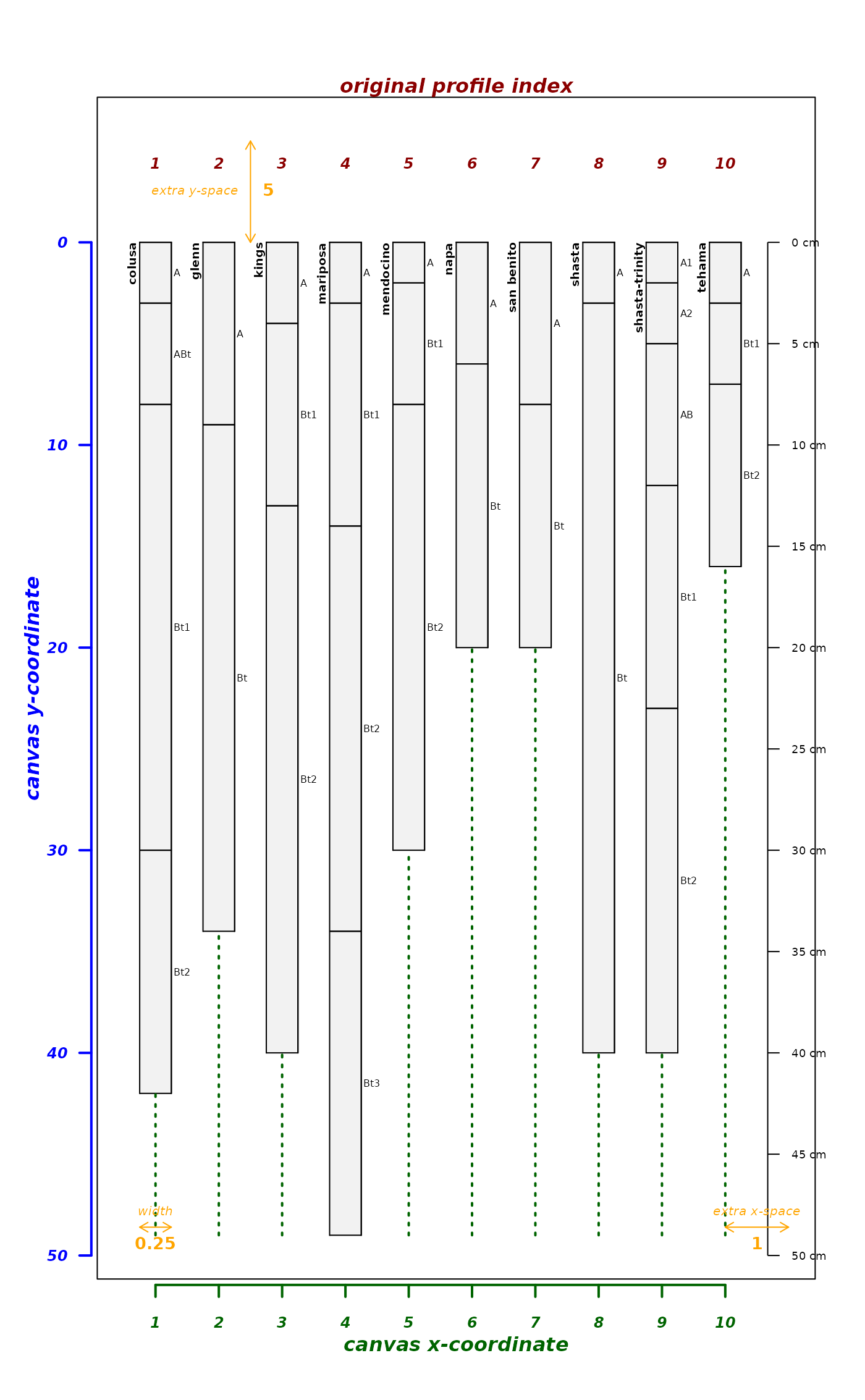# attempt to adjust using defaults
explainPlotSPC(sp4, name='name', relative.pos=fixOverlap(pos))
#> 29 iterations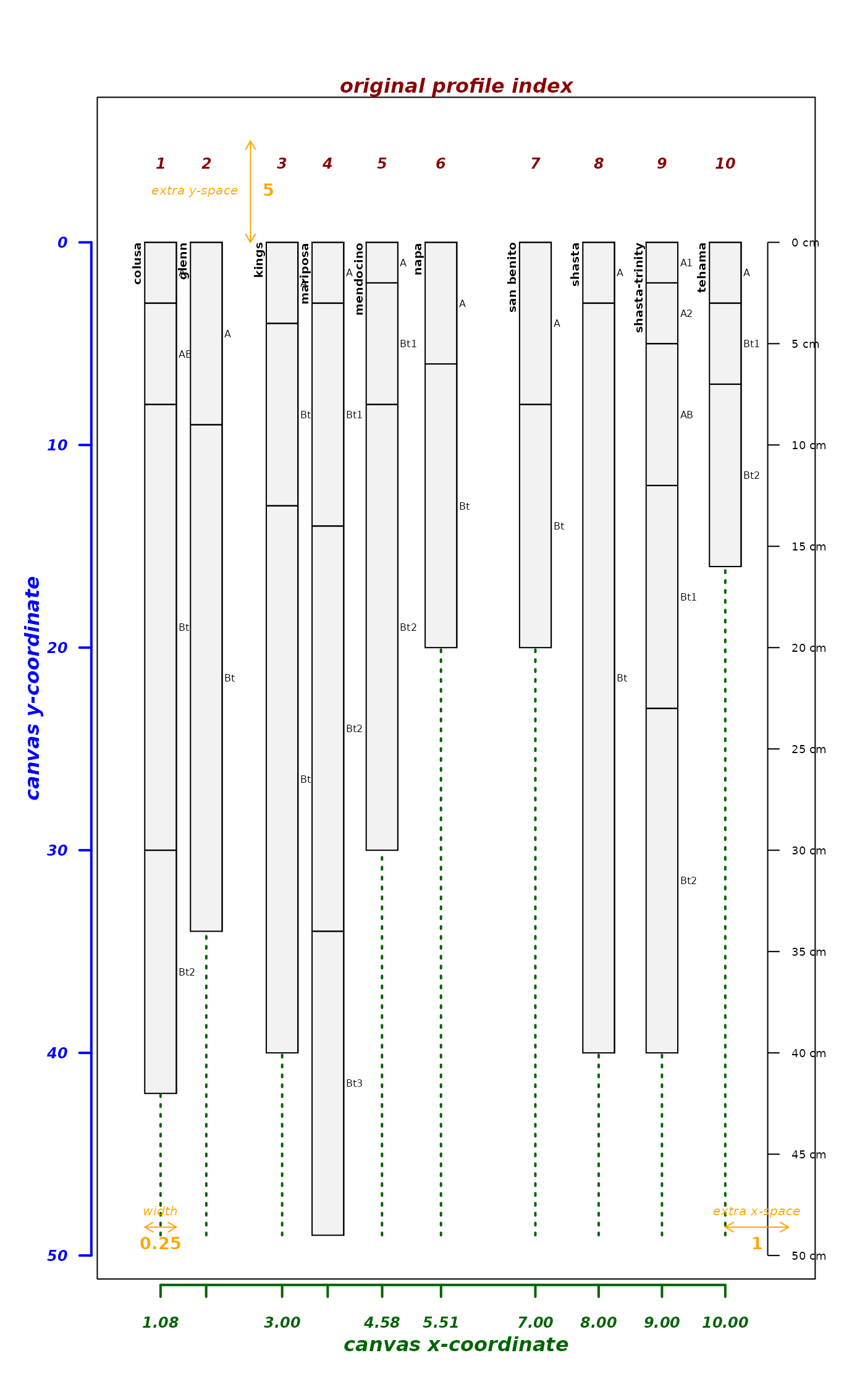# attempt to adjust and tinker with defaults
explainPlotSPC(sp4, name='name', relative.pos=fixOverlap(pos, adj = 0.2))
#> 9 iterations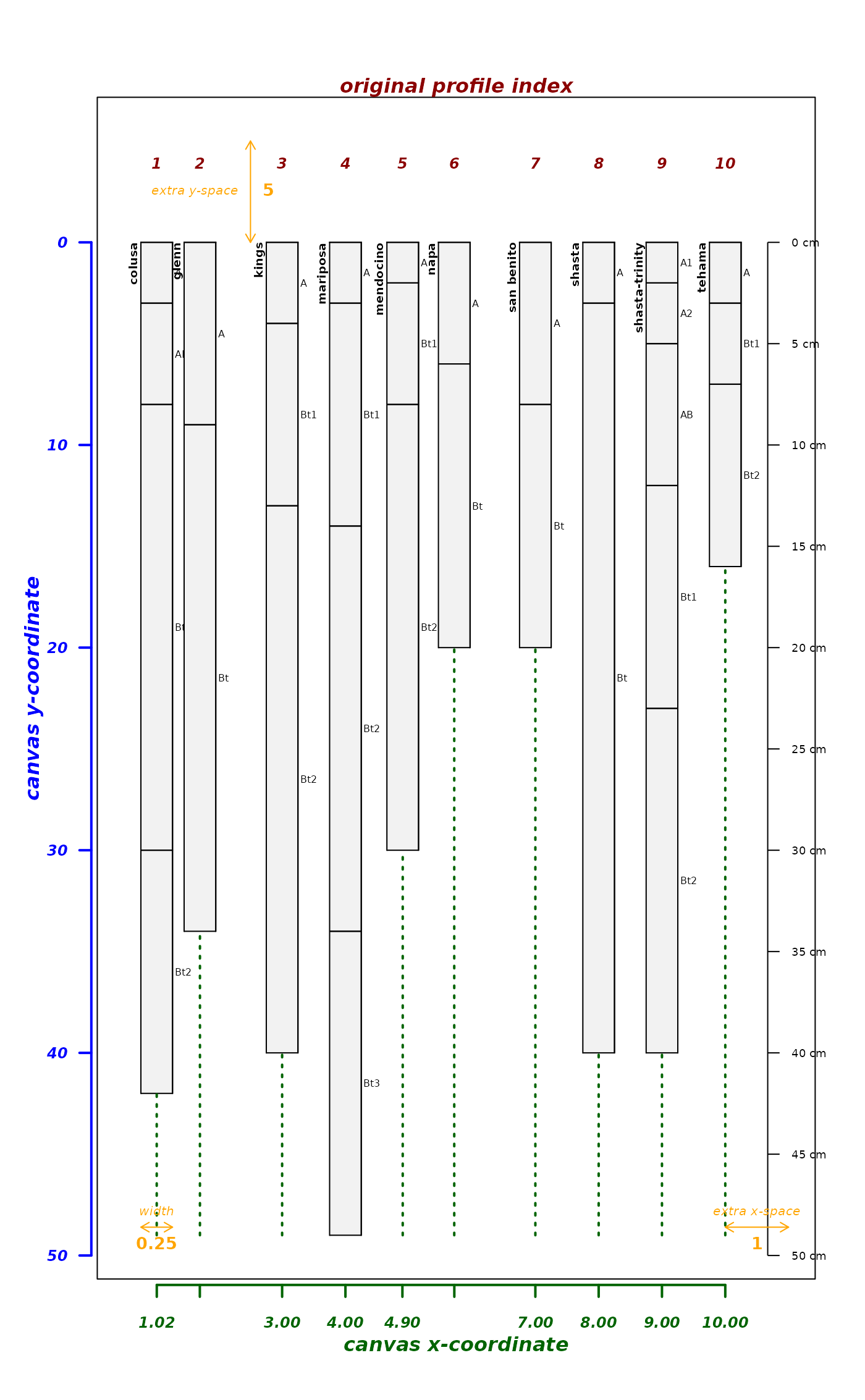set.seed(10101)
explainPlotSPC(sp4, name='name', relative.pos=fixOverlap(pos, thresh = 0.7))
#> 10 iterations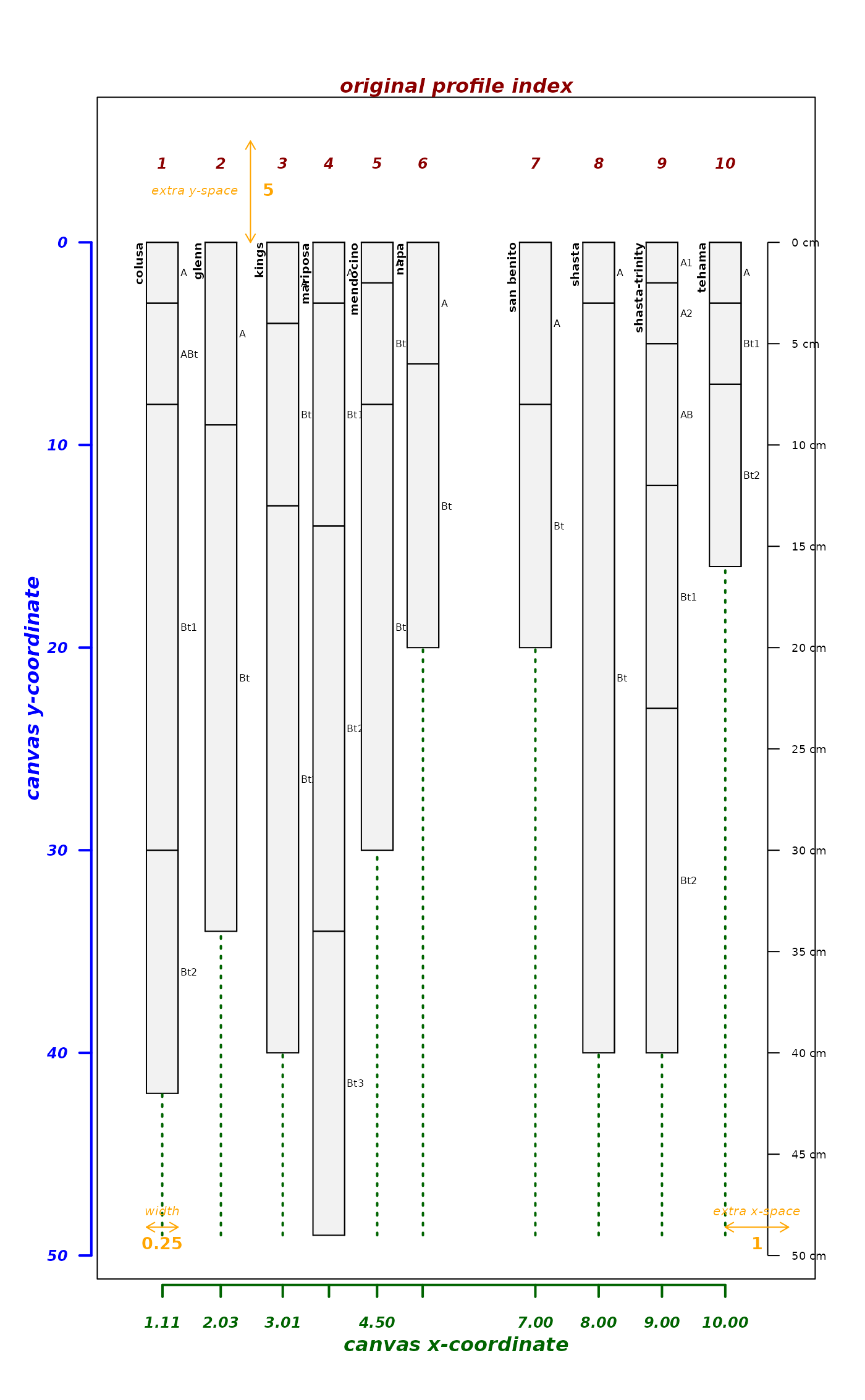pos <- c(1, 2, 3, 3.3, 5, 5.1, 5.5, 8, 9, 9.1)

# tinker
explainPlotSPC(sp4, name='name', relative.pos=pos)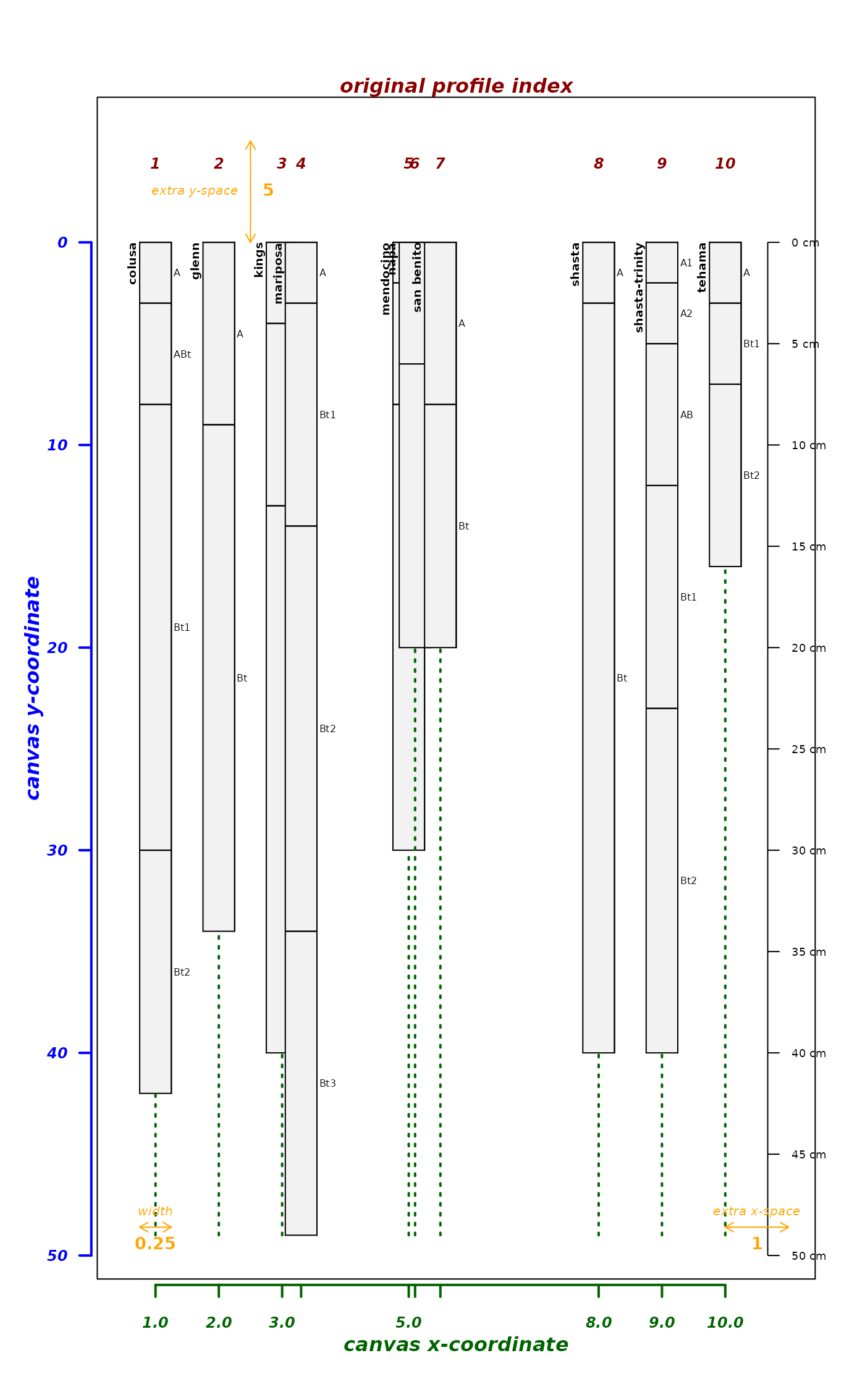explainPlotSPC(sp4, name='name', relative.pos=fixOverlap(pos))
#> 27 iterations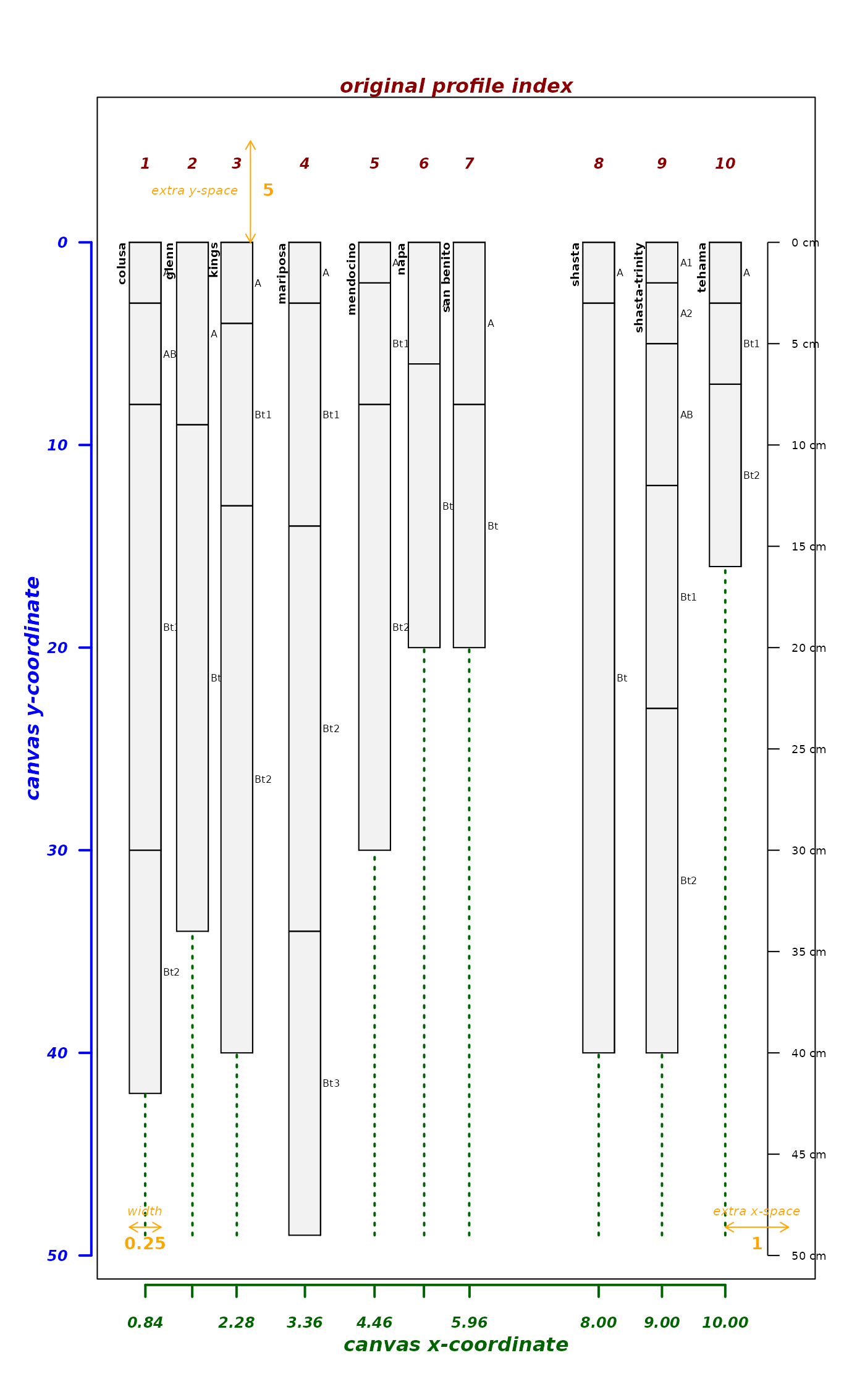explainPlotSPC(sp4, name='name', relative.pos=fixOverlap(pos, thresh = 0.7))
#> 18 iterations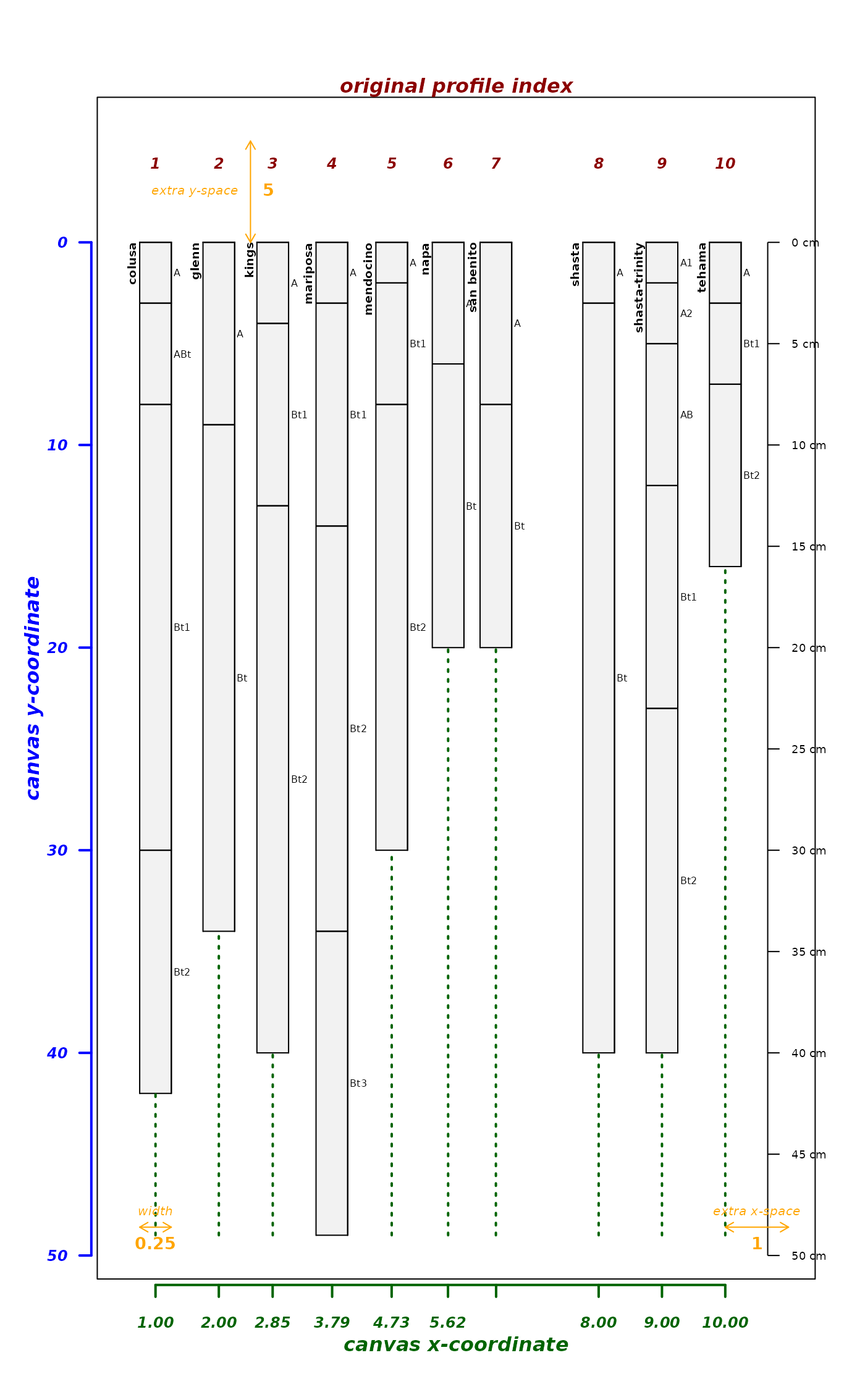explainPlotSPC(sp4, name='name', relative.pos=fixOverlap(pos, thresh=0.7, adj = 0.2))
#> 120 iterations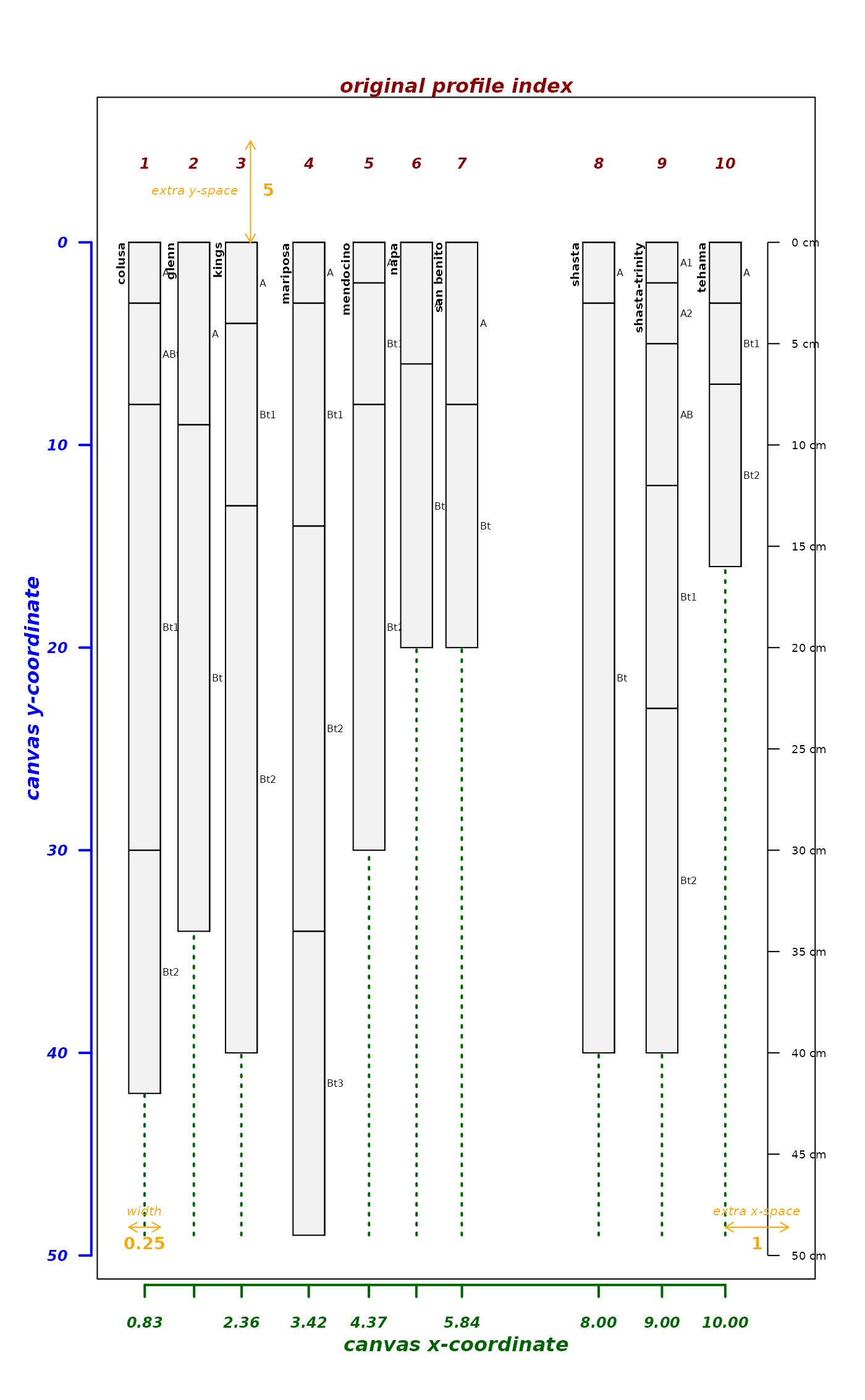# no solution possible given these constraints
explainPlotSPC(sp4, name='name', relative.pos=fixOverlap(pos, thresh=1, adj = 0.2))
#> maximum number of iterations reached, using regular sequence